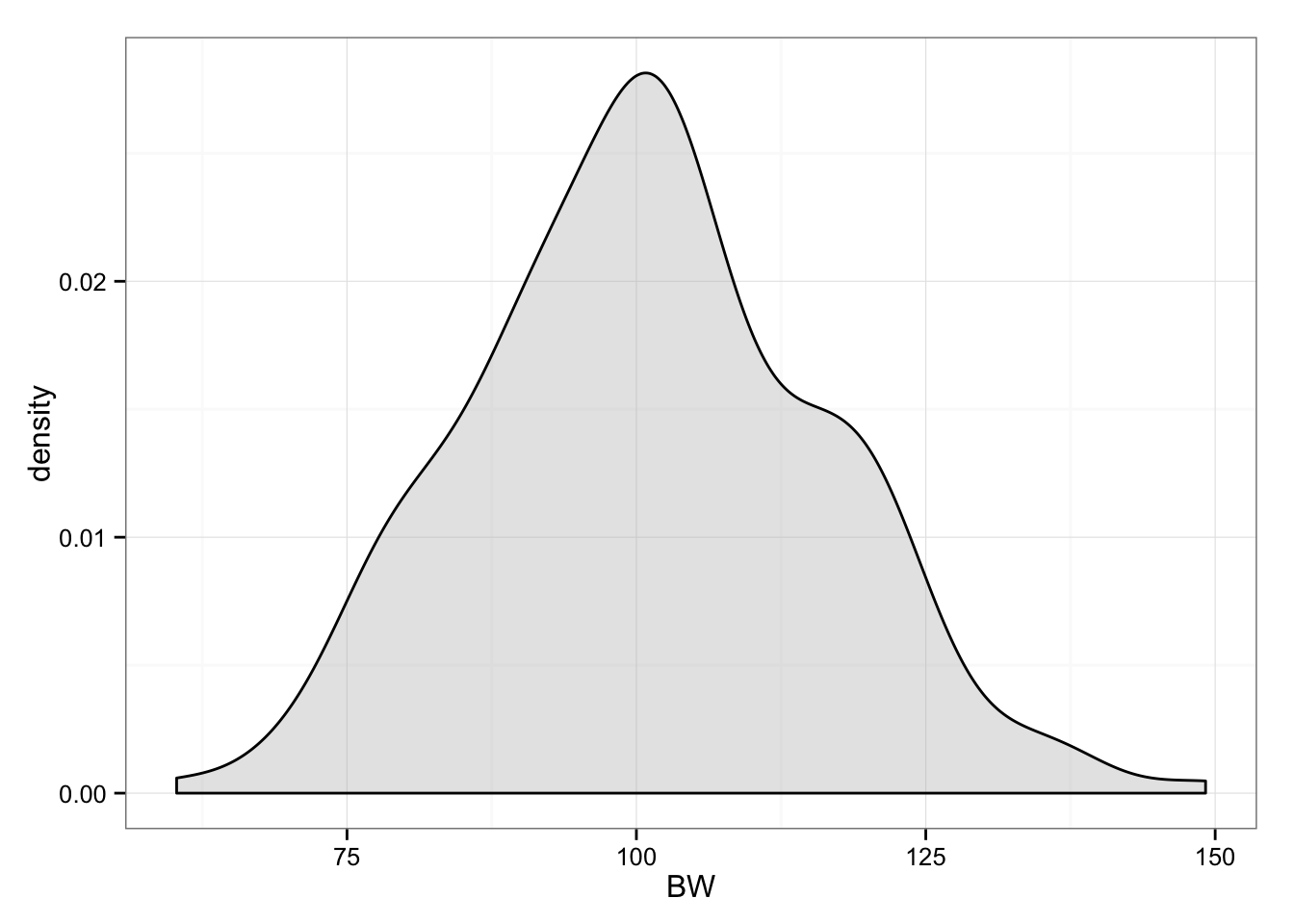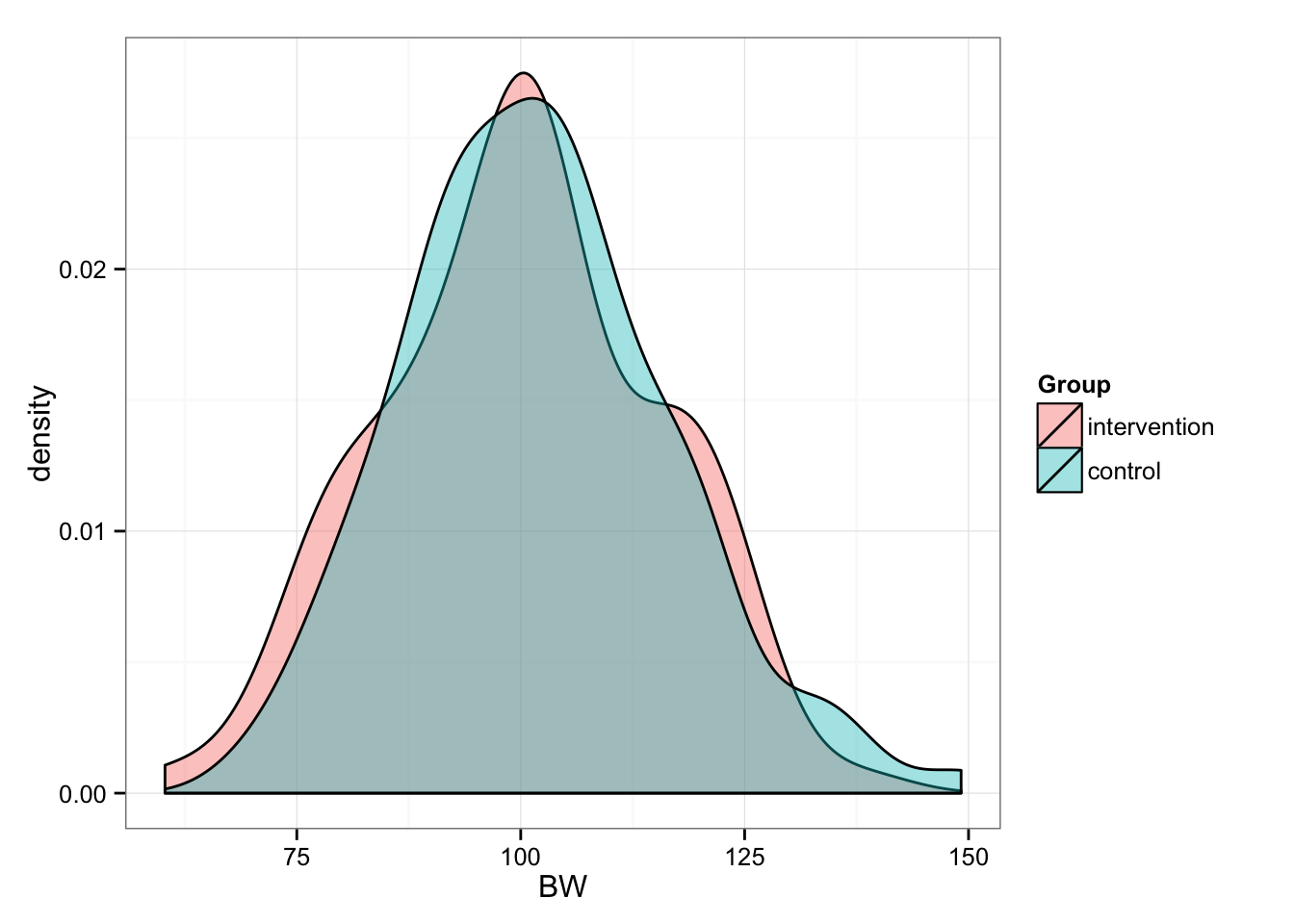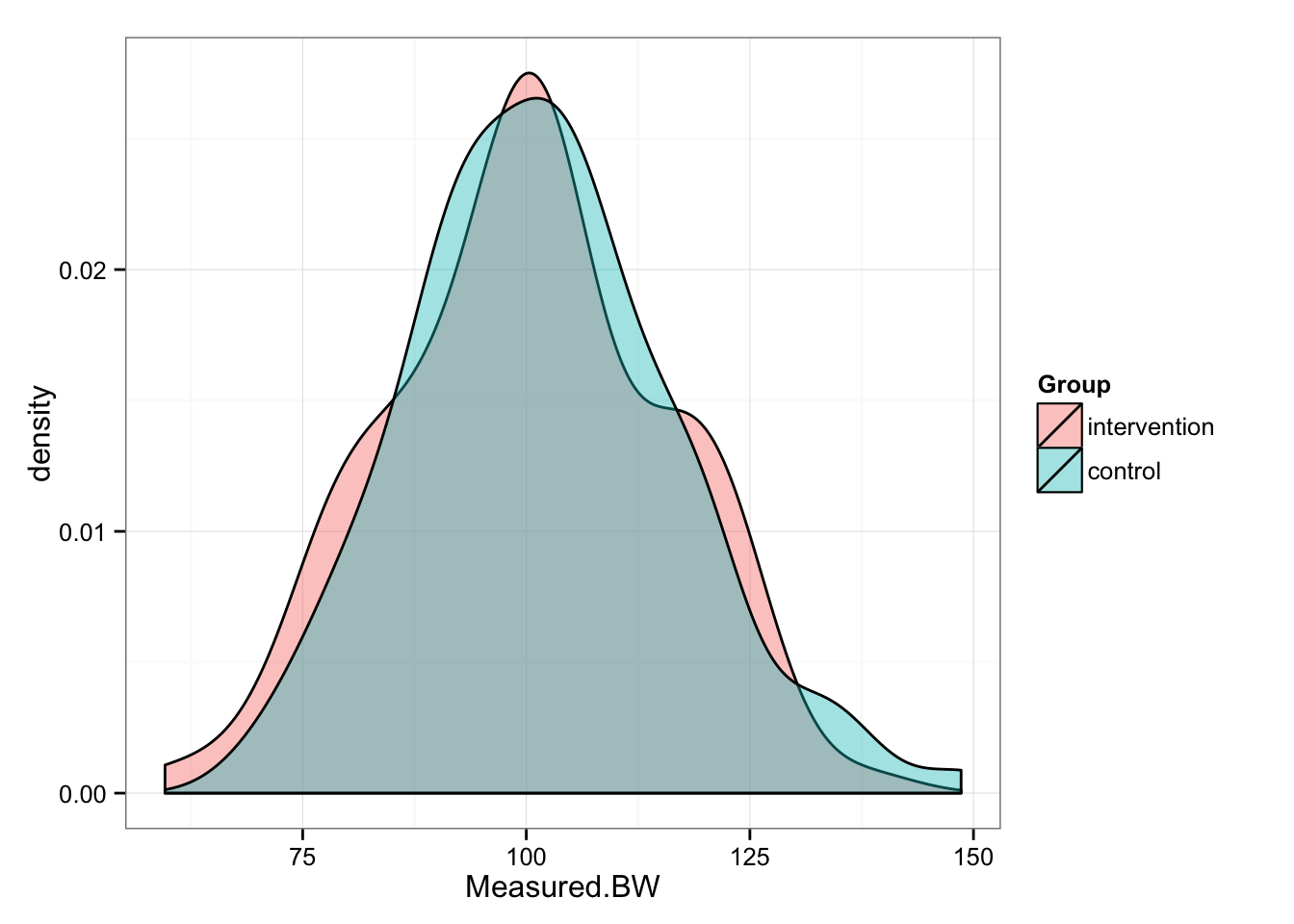## Introduction

Latest obsesion of the researches is individual variation in training responses. The motivation behind this approach (known and emphasized in theory of training as individualization principle) is the creation of personalized medicine or personalized training.

Unfortunately, sometimes we see these individual differences (in treatment reaction), although they are artefacts of within-individual typical variation/error of measurement and regression to the mean.

These are the very important concepts discussed in great paper by Atkinson and Batterham in Journal of Experimental Physiology (2015, ahead of print). I highly urge you to read it before proceeding with the following simulation of mine.

## Problem

Without scaring the non-statistically inclined readers, I will use very simple example from real life. We are interested in effects of one training + diet intervention on body weight BW of our subjects, and whether we can identify the responders vs. non-responders.

If there are responders and non-responders, we might be interested in what other variable can predict it (using mediation/moderation analysis, ANCOVA, mixed-models and so forth - please note that I am not that versed in these yet).

It is important to state, in my opinion there are no general responders and non-responders - they are related to intervention at hand (although there might be individuals who are genetically more lucky in general - hence they response positively to anything thrown at them).

Let’s assume we recruited 200 participants, with “real” body weight of 100kg and around 15kg between-individual SD.

``````library(ggplot2)
library(reshape2)

set.seed(1107) # Set the random number seed so you can replicate the data

n.subjects <- 200

# Generate our sample
pre.subjects.real.BW <- rnorm(mean = 100,
sd = 15,
n = n.subjects)

# Plot histogram
gg <- ggplot(data.frame(BW = pre.subjects.real.BW), aes(x = BW))
gg <- gg + geom_density(fill = "grey", alpha = 0.4)
gg <- gg + theme_bw()
gg``````Now we randomly split them into two groups (n = 100): Intervention and Control

``````# Generate 100 random numbers (uniformly)
group.index <- sample(1:n.subjects, n.subjects / 2)

# Pull out intervention subjects
pre.intervention.real.BW <- pre.subjects.real.BW[group.index]

# Pull out control subjects
pre.control.real.BW <- pre.subjects.real.BW[-group.index]

# Create data frame for plotting
df <- data.frame(intervention = pre.intervention.real.BW,
control = pre.control.real.BW)

# Reshape for ggplot
df <- melt(df,
id.vars = NULL,
variable.name = "Group",
value.name = "BW")

# Plot
gg <- ggplot(df, aes(x = BW, fill = Group))
gg <- gg + geom_density(alpha = 0.4)
gg <- gg + theme_bw()
gg````````````# And summary statistics
summary(pre.intervention.real.BW)``````
``````##    Min. 1st Qu.  Median    Mean 3rd Qu.    Max.
##   60.28   89.62  100.30  100.00  110.50  138.00``````
``summary(pre.control.real.BW)``
``````##    Min. 1st Qu.  Median    Mean 3rd Qu.    Max.
##   69.56   92.16  101.50  101.80  110.20  149.20``````

Now we have two equal groups of n = 100, BW = 100 (15). But before we proceed, let’s deal with “real” BW. In this case “true” refers to “true” bodyweight of the subject. But as we know any measure varies because of measurement error and biological variability (i.e. noise). For example, if my “real” bodyweight is 95kg, due normal biological fluctuations and my scale error I might score 94-96kg on different days.

Let’s assume (we should measure the reliability of our metrics, but for the sake of this simulation we assume) that our typical variation is 0.5 kg and it is normally distributed. This means that in 95% of times, any single subject weight will fluctuate between -1 and +1 kg.

So let’s apply this typical variation to our pre- bodyweights.

``````# Create a simple function that creates typical variation
TV <- 0.5
typical.var <- function(SD = TV, n = n.subjects / 2) {
return(rnorm(mean = 0, sd = SD, n))
}

# Add typical variation or noise
pre.intervention.measured.BW <- pre.intervention.real.BW + typical.var()
pre.control.measured.BW <- pre.control.real.BW + typical.var()

# Create data frame for plotting
pre <- data.frame(intervention = pre.intervention.measured.BW,
control = pre.control.measured.BW)

# Reshape for ggplot
pre <- melt(pre,
id.vars = NULL,
variable.name = "Group",
value.name = "Measured.BW")

# Plot
gg <- ggplot(pre, aes(x = Measured.BW, fill = Group))
gg <- gg + geom_density(alpha = 0.4)
gg <- gg + theme_bw()
gg````````````# And summary statistics
summary(pre.intervention.measured.BW)``````
``````##    Min. 1st Qu.  Median    Mean 3rd Qu.    Max.
##   59.60   89.35  100.40  100.00  110.30  138.10``````
``summary(pre.control.measured.BW)``
``````##    Min. 1st Qu.  Median    Mean 3rd Qu.    Max.
##   69.46   92.10  101.40  101.90  110.30  148.60``````

We can perform t test to confirm that our groups in pre- conditions are identical

``````t.test(pre.intervention.measured.BW,
pre.control.measured.BW)``````
``````##
##  Welch Two Sample t-test
##
## data:  pre.intervention.measured.BW and pre.control.measured.BW
## t = -0.883, df = 197.918, p-value = 0.3783
## alternative hypothesis: true difference in means is not equal to 0
## 95 percent confidence interval:
##  -6.063432  2.312780
## sample estimates:
## mean of x mean of y
##   99.9953  101.8706``````

Let’s assume that the “real” change in Intervention group is 3 kg - in other words all subjects that were in intervention group reduced their “real” BW for 3 kg. In this case 3 kg represents smallest worthwhile change (SWC).

The control group experienced 0 (zero) change in “real” bodyweight, hence no effect.

But, since we are measuring post- bodyweight as well, we are again introducing typical variation. Let’s calculate post- real and measured bodyweight for both groups

``````# the "real" change of 3 kg in intervention group
post.intervention.real.BW <- pre.intervention.real.BW - 3

# No "real" change in control group
post.control.real.BW <- pre.control.real.BW - 0

# Now let's calculate "measures" post- bodyweights by adding typical variation
post.intervention.measured.BW <- post.intervention.real.BW + typical.var()
post.control.measured.BW <- post.control.real.BW + typical.var()

# Create data frame for plotting
post <- data.frame(intervention = post.intervention.measured.BW,
control = post.control.measured.BW)

# Reshape for ggplot
post <- melt(post,
id.vars = NULL,
variable.name = "Group",
value.name = "Measured.BW")

# Plot
gg <- ggplot(post, aes(x = Measured.BW, fill = Group))
gg <- gg + geom_density(alpha = 0.4)
gg <- gg + theme_bw()
gg``````